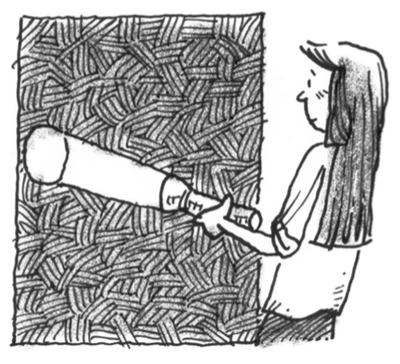### Home > APCALC > Chapter 10 > Lesson 10.1.6 > Problem10-74

10-74.

Mariah walks towards a wall with a flashlight beaming straight ahead. When she is $5$ feet away from the wall, the radius of the circle of light is $2$ feet. If she walks at a rate of $0.5$ ft/sec, calculate the rate at which the diameter of the circle of light is changing.

The radius is directly proportional to Mariah's distance from the wall. That is, $d = kr$.

$r=\frac{2}{5}d$

Differentiate:

$\frac{dr}{dt}=\frac{2}{5}\cdot\frac{dd}{dt}$

$\frac{dd}{dt}=-0.5$